Checkout JEE MAINS 2022 Question Paper Analysis : Checkout JEE MAINS 2022 Question Paper Analysis :

# JEE Advanced Maths Area Under the Curves Previous Year Questions with Solutions

Area under the curves JEE Advanced previous year questions with solutions are given on this page. These solutions are designed by our team of experts. The area under a curve between two points is calculated by doing a definite integral between the two points. Students can expect questions from this topic for any entrance exam. So, students are advised to revise these solutions so that they can easily crack the JEE Advanced exam.

Question 1: Area of the region bounded by the curve y = ex and lines x = 0 and y = e is

(a) e-1

(b) 1

(c) e – ∫01ex dx

(d) ∫1e ln y dy

Solution: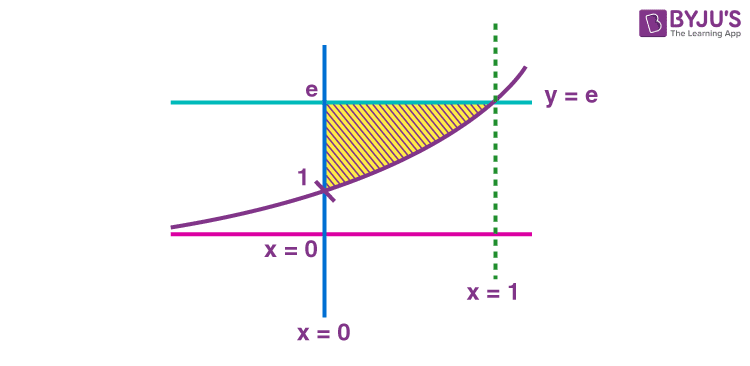Required area is A = ∫1e ln y dy = e – ∫01 ex dx

= e – (ex)01

= e – (e-1)

= 1

Hence option b, c, d are correct.

Question 2: A farmer F1 has a land in the shape of a triangle with vertices at P(0, 0), Q(1, 1) and R(2, 0). From this land, a neighbouring farmer F2 takes away the region which lies between the side PQ and a curve of the form y = xn (n > 1). If the area of the region taken away by the farmer F2 is exactly 30% of the area of ΔPQR, then the value of n is

Solution: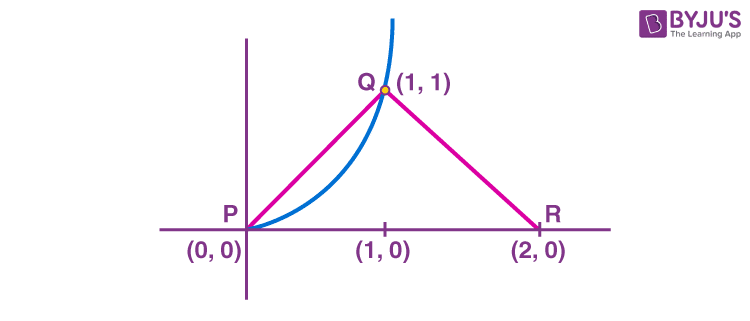Area of ΔPQR = ½ bh = ½ × 2×1

= 1 unit

Given that area of the region taken away by the farmer F2 is exactly 30% of the area of ΔPQR.

So area taken away by F1 = 0.3

So ∫01 (x-xn)dx = 0.3

=> [x2/2 – xn+1/n+1)]01 = 0.3

=> [½ – 1/(n+1) = 0.3

=> 0.2 = 1/(n+1)

=> 2n+2 = 10

=> 2n = 8

=> n = 4

Question 3: If the line x = α divides the area of region R = {(x, y) ∈ R2, x3 ≤ y ≤ x, 0 ≤ x ≤ 1} into two equal parts, then

(a) ½ < α < 1

(b) α4 + 4α2 – 1 = 0

(c) 0 < α ≤ ½

(d) 2α4 – 4 α2 + 1 = 0

Solution: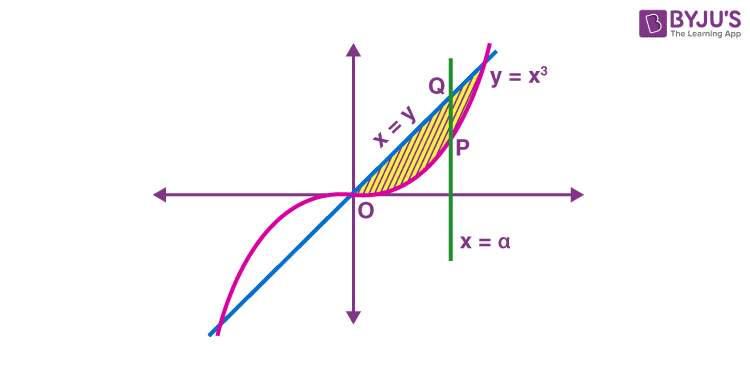Required area is the area of the shaded portion.

A = ∫01 (x-x3) dx

= [x2/2 – x4/4]01

= ½ – ¼

= 1/4

Given that line x = α divides the area in 2 equal parts,

Area (OPQ) = A/2 = 1/8

A/2 = ∫0α (x-x3) dx

= [x2/2 – x4/4]0α

=> (α2/2) – (α4/4) = ⅛

=> 2α4 – 4α2 + 1 = 0

=> (α2-1)2 = 1/2

=> α2 = (√2-1)/√2

=> ½ < α < 1

Hence option a and d are correct.

Question 4: The area (in sq.units) of the region {(x, y): 0 ≤ y ≤ x2+1, 0≤y≤x+1, ½ ≤ x≤ 2} is

(a) 23/16

(b) 79/24

(c) 79/16

(d) 23/6

Solution: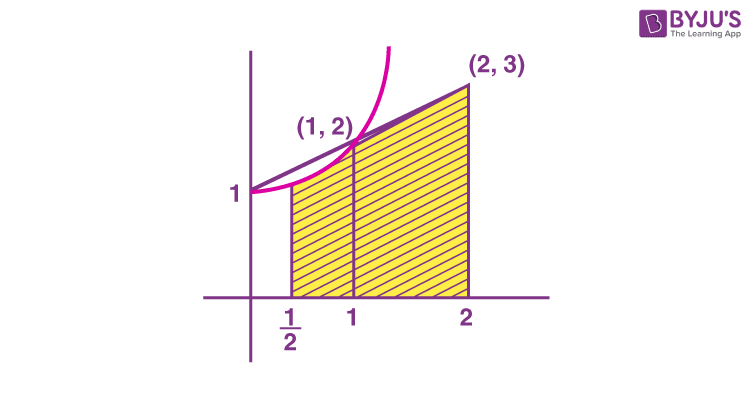Required area = ∫1/21 (x2+1) dx + ∫12 (x+1) dx

= [x3/3 + x]11/2 + [x2/2 + x]12

= [4/3 – 13/24] + 5/2

= (32 – 13 + 60)/24

= 79/24

Hence option b is the answer.

Question 5: Let the functions f: R→ R and g: R→ R be defined by f(x) = ex-1 – e-|x-1| and g(x) = ½ (ex-1 + e1-x). Then the area of the region in the first quadrant bounded by the curves y = f(x), y = g(x) and x = 0 is

(a) (2 – √3) + ½ (e – e-1)

(b) (2 + √3) + ½ (e – e-1)

(c) (2 – √3) + ½ (e + e-1)

(d) (2 +√3) + ½ (e + e-1)

Solution:

Given that f(x) = ex-1 – e-|x-1|

So

$$\begin{array}{l}f(x)= \left\{\begin{matrix} 0 & x\leq 1\\ e^{x-1}-e^{1-x}& x\geq 1 \end{matrix}\right.\end{array}$$

g(x) = ½ (ex-1 + e1-x)

If f(x) = g(x)

=> ex-1 – e-(x-1) = (ex-1+e1-x)/2

=> e2(x-1) = 3

=> x = ½ ln 3 + 1

=> x = 1+ ln √3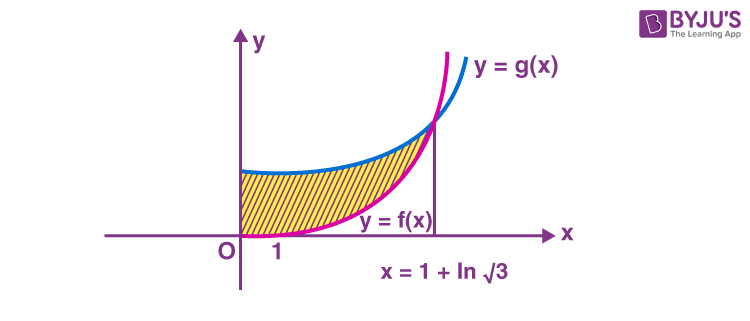Bounded area = ∫0(1/2)ln3 + 1g(x) dx – ∫1(1/2)ln3 + 1f(x) dx

= ½ [ex-1 – e1-x]o(1/2)ln3 + 1 – [ex-1+e1-x]1(1/2)ln3 + 1

= (2 – √3) + ½ (e – e-1)

Hence option a is the answer.

Question 6: If the area (in sq.units) bounded by the parabola y2 = 4λx and the line y = λx, λ >0 is 1/9, then λ is equal to

(a) 2√6

(b) 48

(c) 24

(d) 4√3

Solution:

Given parabola y2 = 4λx

And line y = λx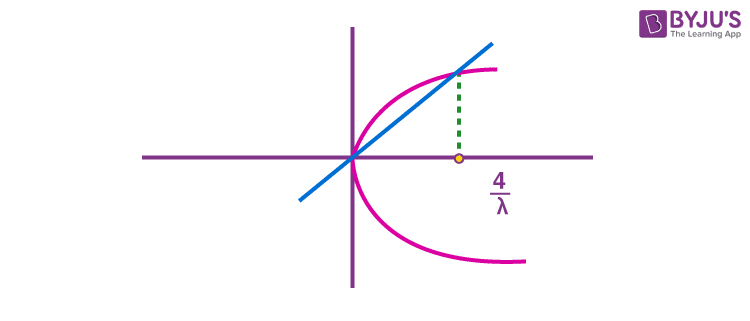Putting y = λ in y2 = 4λx,

x = 0, 4/λ

Area = ∫04/λ (√(4λx) – λx) dx = 1/9

=> 2√λ (x3/2/3/2)04/λ – λ(x2/2)04/λ = 1/9

Solving we get λ = 24

Hence option c is the answer.

Question 7: The area (in sq.units) of the region bounded by the curves y = 2x and y = |x+1|, in the first quadrant is

(a) loge 2 + (3/2)

(b) 3/2

(c) 1/2

(d) (3/2) – 1/loge2

Solution: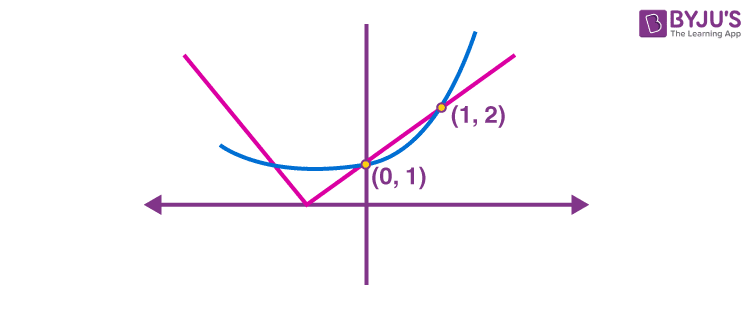Area = ∫01 ((x+1) – 2x)dx

= [x2/2 + x – 2x/ln 2]01

= (½ + 1 – 2/ln 2) – (-1/ln 2)

= (3/2) – 1/ln 2

Hence option d is the answer.

Question 8: The area (in sq.units) of the region A = {(x, y): y2/2 ≤ x ≤ y+4} is

(a) 53/3

(b) 30

(c) 16

(d) 18

Solution: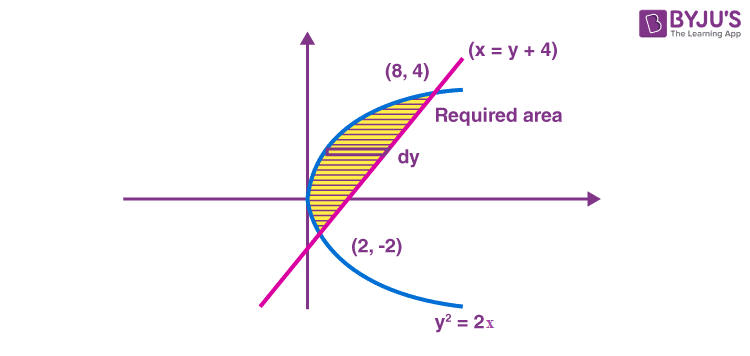Given A = {(x, y): y2/2 ≤ x ≤ y+4}

Hence area = ∫-24 x dy

= ∫-24 (y+4- (y2/2)) dy

= [(y2/2) + 4y – y3/6]-24

= (8 + 16 – 64/6) -(2 – 8 + 8/6)

= 108/6

= 18

Hence option d is the answer.

Question 9: The area (in sq.units) of the region bounded by the parabola, y = x2 + 2 and the lines, y = x+1, x = 0, and x = 3 is

(a) 15/4

(b) 21/2

(c) 17/4

(d) 15/2

Solution: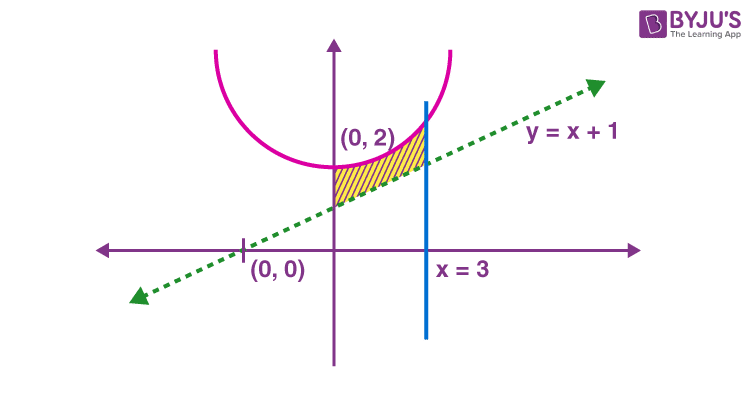Area of the bounded region = ∫03 [(x2+2) – (x+1)] dx

= [x3/3 – x2/2 + x]30

= 9 – 9/2 + 3

= 15/2

Hence option d is the answer.

Question 10: The area of the region {(x, y): xy ≤8, 1≤y≤x2} is

(a) 8 loge2 – 14/3

(b) 16 loge2 – 14/3

(c) 8 loge2 – 7/3

(d) 16 loge2 – 6

Solution: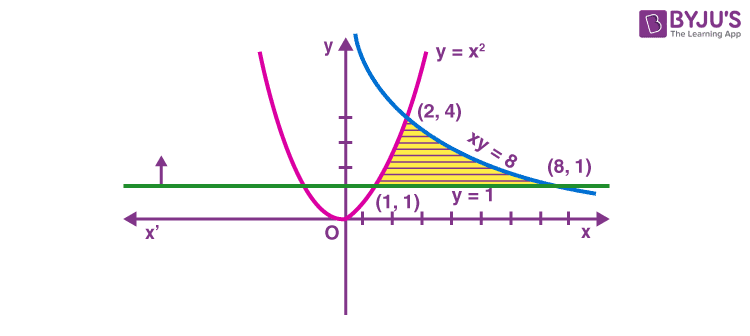Given xy ≤ 8, 1 ≤ y ≤ x2

Intersection points of xy = 8 and y = 1 is (8, 1): xy = 8 and y = x2 is (2, 4) and y = x2 and y = 1 is (1, 1).

Required area = ∫12 x2 dx + ∫28 (8/x) dx – ∫18 dx

= [x3/3]12 + [8 ln x]28 – [x]18

= (8/3) – (⅓) + 8 ln 8 – 8 ln 2 – (8-1)

= (7/3) + 24 ln 2 – 8 ln 2 – 7

= 16 ln 2 – 14/3

Hence option b is the answer.

Recommended Video